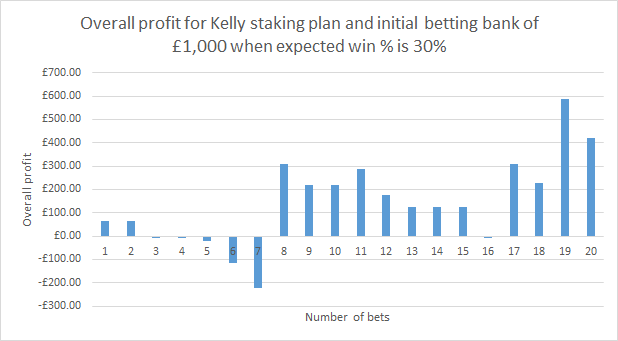# Kelly staking plan

Kelly staking plan is using mathematical formula to determine optimal stake for your bets. It requires that you know your winning percentage and odds at which bet will be placed.

Original Kelly formula is f = (b * p - q) / b

• f is the percentage of your betting bank that should be used as stake
• b is the net price at which bet will be placed
• p is probability of winning e.g. your expected win percentage, you can check this at each tipster profile page
• q is the probability of losing, that is 1 - pWe can rewrite this formula like this

f = (b * p - q) / b = (b * p - (1 - p)) / b = (b * p - 1 + p) / b = (p * (b + 1) - 1) / b

For example, if your winning percentage (p) is 30% and price at which you are betting is 3.5 then f value will be calculated like this:

f = (0.30 * (2.5 + 1.0) - 1.0) / 2.5 = (0.30 * 3.5 - 1.0) / 2.5 = (1.05 - 1.0) / 2.5 = 0.05 / 2.5 = 0.02

This means that we need to invest 2% of our betting bank. If betting bank is £1,000 then our bet size will be £20.0

Optionally you can also use divisor and divide f if you wish to use smaller amount of your betting bank as bet size. If we used divisor of 4 then our bet size would be £20.0 / 4 = £5.00

You can download worksheet for Kelly staking plan that will allow you to set your own settings and see the results.

For more details on Kelly criterion you should visit Kelly criterion Wikipedia page.